# Exploring Probabilistic Data Structures: Bloom Filters

0
3907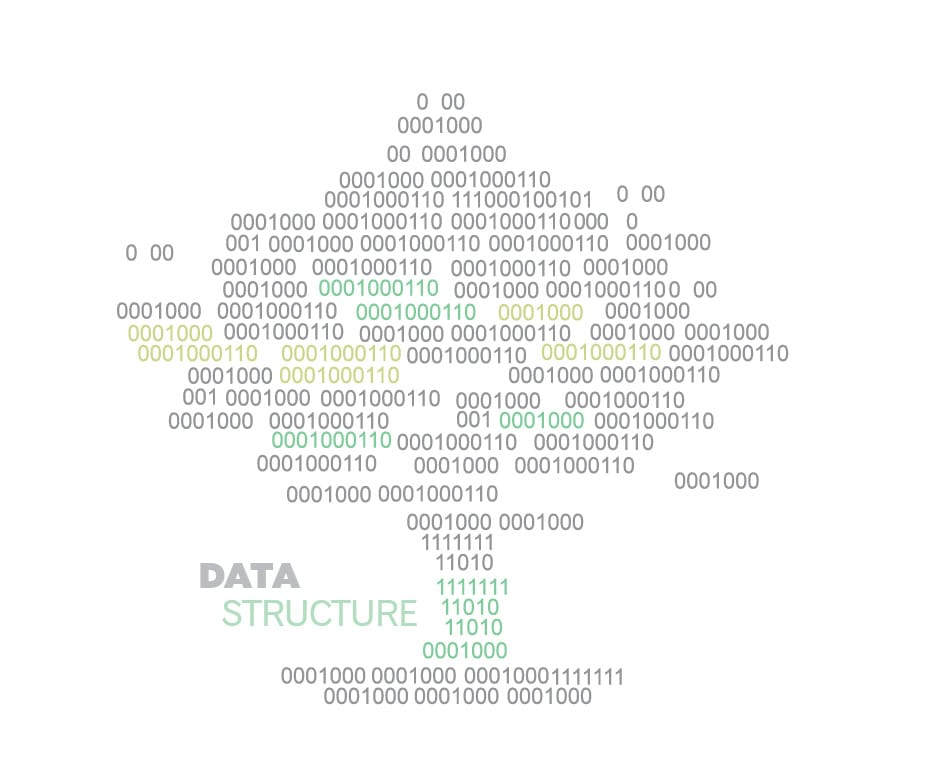Bloom filters, conceived by Burton Howard Bloom in 1970, are probabilistic data structures that are used to test whether an element belongs to a set or not.

Processing a large set of entities becomes an expensive and tedious task, particularly for set operations, such as the union and intersection of elements in a particular set. An example would be the processing of a very large set of numbers (e.g., 100 million) and executing a query to determine whether a number belongs to the group or not. The naive method of executing such a query is by iterating over all set members. However, this is expensive, consumes precious CPU cycles and also pollutes the cache memory.

Probabilistic data structures elegantly solve such problems with minimal resources and with an acceptable error rate. They outperform regular solutions like HashMaps and HashTables, which have large memory footprints. In this article, we will discuss one such data structure—the Bloom filter. It is one of the most popular data structures due to its simplicity of implementation, small memory footprint, and efficiency. It is used for request filtering over a large set of data, and used by Web browsers such as Chrome, Web caches of Akamai, and websites like Quora and Medium.

What is a Bloom filter

A Bloom filter was invented by a scientist called Burton Howard Bloom in 1970, to test whether or not an element belongs to a set. The principal property of a Bloom filter is based on probability, in which a positive response of the filter means that the given element could belong to the set. However, a negative response guarantees that the element is not present in the set. The terminology for the scenario in which an element is present in the set but does not return ‘True’ is termed a false positive or the error rate of the filter.

Defining a Bloom filter

Assume we have an input set S = [ x1, x2,…xn ] of n elements. A Bloom filter B can be represented by an array of m bits (zero-initialised). The size of Bloom filter is a major factor influencing its error rate. A large size filter usually has a lower error rate.

`B = [ b1, b2 ...bm-1, bm ]`

A Bloom filter uses k independent hash functions ​​h1…hk that are assumed to be uniformly distributed over the range 1. …m.

A standard Bloom filter has two operations defined as follows:

`1 ≤ i ≤ k B [hi(x)]=1 x ∈S`
• (x) – Check if x is in the Bloom filter. For
`1 ≤ i ≤ k ifB[hi(x)]=1 returnTrue, else return False.`

To represent set S by a Bloom filter B, we need to process each member of S with add function. Here is a simple example to understand the operations of Bloom filter.

• For an input set, S = [1,2,3,4]
• Number of hash functions, k=2 ;
• Size of Bloom Filter, m=8 ;
• We have two hash functions: h1,h2

Bloom filter preparation

The preparation phase involves setting an entry using the add operation in the Bloom filter corresponding to a set member. The add operation maps the input element using the two hash functions. Each hash function output is an index into the Bloom filter array B. After calculating the index, it is set to one. This process will be repeated for all the input entries. The add operation is defined as follows:

```add(x):

i = h1(x)

j = h2(x)

B[i] = 1

B[j] = 1```

The Bloom filter bit array is as follows:

 0 1 2 3 4 5 6 7 0 0 0 0 0 0 0 0
```add (2)

h1(2) = 6

h2(2) = 7```
 0 1 2 3 4 5 6 7 0 0 0 0 0 0 1 1
```add (3)

h1(3) = 4

h2(3) = 1```
 0 1 2 3 4 5 6 7 0 1 0 0 1 0 1 1

To determine the membership of an element in an input set S, the check operation is used. This operation is defined as follows:

```bool check(x):

i = h1(x)

j = h2(x)

return i && j```

The input value is subjected to the same hash functions, and the corresponding bits at the indices are checked if they are set. If all the values are set, then the input value might be present in the set. This is always probable, but not certain because the bits on the positions in question might be set by other add operations of other elements.

When the filter returns False for a given input, it is certain that the input element does not exist in the set. The condition of a false return will occur when one or more hashed indices have zero value. No add operation ever sets those positions to be 1, and hence the input element is not present in the set.

```check (7)

h1(3) = 4

h2(3) = 0```
 0 1 2 3 4 5 6 7 0 1 0 0 1 0 1 1

In the above operation, since a check would return False, the element is definitely not present in the set. This is the utility of the Bloom filter, which eliminates spurious lookup operations.

```check (5)

h1(3) = 6

h2(3) = 7```
 0 1 2 3 4 5 6 7 0 1 0 0 1 0 1 1

In the operation given above, since the check returns True, it is possible that 5 is present in the set. This is a false positive result and an error of the filter.

The error rate of a Bloom filter

The expected value of false positive probability p decides the optimal values for Bloom filter size m, and the number of hash functions k, for an input list of elements. We assume that all the k hash functions are uniform and will choose all m positions in the bit array B of Bloom Filter with equal probability of

The probability of choosing a position among m positions is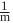. The probability of not choosing a position is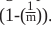Since there are k hash functions, the probability of not choosing that position for a position is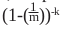.

For n entries, we repeat the above process n times and get the probability of not setting that position as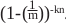. So, the probability that the position is set after the above process is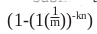. Considering all the positions that are set, the final probability we get is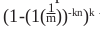The effectiveness of the Bloom filter depends on the values of p, k, n and m. We need to find optimal values of m and k depending on the input values of p and n. By the use of differential calculus, we get the following results, for optimal values.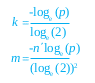Experimental results

The Bloom filter technique is used by Web browsers to filter malicious URLs. It helps to solve the problem of fast lookup from a list of such URLs. A browser has to get the information from a remote server, without a local lookup solution, which is slow and expensive. One solution is to use a HashTable to store these URLs. But HashTables have large memory requirements for huge lists such as those containing 10 million entries, which need 250MB to 300MB of memory.

A Bloom filter effectively handles such problems and offers constant time O(1) operations while using negligible memory. A Bloom filter of a size of one million entries would need only 1MB of memory. An input URL is checked in the Bloom filter embedded in the browser. If the filter returns False, then one can be sure that the URL is not present in the list of malicious sites, since the false negative case is not possible in a Bloom filter. If the filter returns True, the input URL could or could not be present in the malicious URL list. So the browser has to make a remote call to determine the validity of the URL.

The Bloom filter is very useful especially when the input search is a spurious value because the filter will identify it as ‘not present’ in the target set. This reduces the load of remote calls and decreases latency of the browser lookup operations.

We ran an experiment in which there was a list of one million malicious URLs generated randomly. The incoming URL was checked against the malicious URL list using a Bloom filter. We found that the Bloom filter successfully identified 88 per cent of malicious URLs. The code for the Bloom filter implementation and the test experiment is available at GitHub (https://github.com/souravchk/Algorithms-and-Data-Structures/tree/master/bloom-filters).